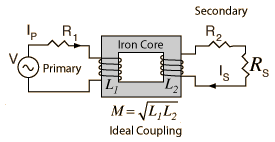# Transformer Power Calculation

For a supply voltage of volts
at Hz and a primary inductance
 L1 = 1 Henry.
 Primary turns: NP =
 Secondary turns: NS=
 Secondary voltage: VS=voltsAssuming identical geometry, the secondary inductance L2 = H.
 Assuming ideal coupling, mutual inductance M = H
 Primary winding resistance R1= Ω
 Secondary winding resistance R2 = Ω
With the above specified parameters, the calculated values are:
 Effective primary resistance RP=Ω
 Effective primary reactance XP = Ω
 Primary impedance ZP =Ω at °
 Secondary impedance Z2 = Ω at °
 The power dissipated in the primary winding = W = % of the power.
 The power dissipated in the secondary winding = W = % of the power.
 The power delivered to the load = W = % of the power.
Notes: Values may be entered for any of the transformer parameters. Default values will be entered for non-specified values, but they can be changed as part of the exploration. Click outside any data field to initiate the calculation.
 Numerical example Plot of typical results
 Discussion of loaded transformer Circuit equations
Index

Transformer concepts